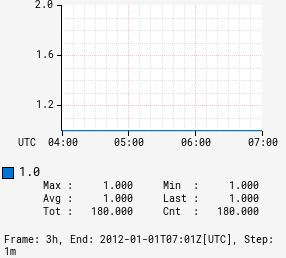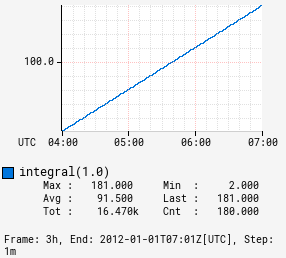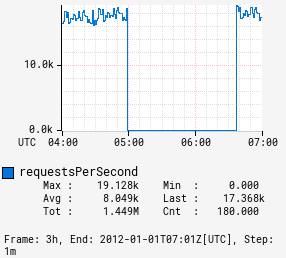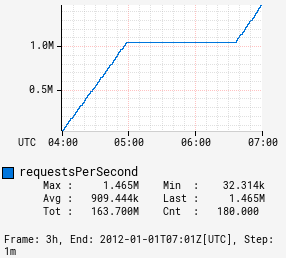# integral

Input Stack:
 TimeSeriesExpr
Output Stack:
 TimeSeriesExpr

Sum the values across the evaluation context. This is typically used to approximate the distinct number of events that occurred. If the input is non-negative, then each datapoint for the output line will represent the area under the input line from the start of the graph to the time for that datapoint. Missing values, `NaN`, will be treated as zeroes. For example:

Input :integral
0 0
1 1
-1 0
NaN 0
0 0
1 1
2 3
1 4
1 5
0 5

For a counter, each data point represents the average rate per second over the step interval. To compute the total amount incremented, the value first needs to be converted to a rate per step interval. This conversion can be performed using the :per-step operation.

Examples:

 Before After```1 ``` ```1,:integral ```
 Before After```name,requestsPerSecond,:eq, :sum, :per-step ``` ```name,requestsPerSecond,:eq, :sum, :per-step, :integral ```